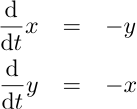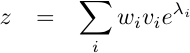# Lanchester’s Law: why small advantages swell in StarCraft

StarCraft and StarCraft II are very popular real time strategy games. The core of these games is the mining of resources, and conversion of those resources into specialized military units. Idealized fighting and predator/prey relations have long been analyzed in terms of differential equations. We use the differential equation formalism (in particular Lanchester’s equations of 1916) to discuss expected game outcomes and how, in principle, one can derive a StarCraft strategy that complements search, simulation or more classic artificial intelligence techniques.StarCraft doesn’t match any of the definitions of a “proper mathematical game.” StarCraft includes non-simultaneous moves, hidden information and delayed results (construction ends some time after it is started). So the game does not fit directly into von Neuman and Morgenstern’s zero-sum payoff matrix game theory. The hidden information and lack of a formal turn structure also prevent StarCraft from fitting in the Berlekamp, Conway and Guy combinatorial game formalism. But we can apply some of their ideas on valuation and seperability.

To analyze we must first settle on some kind of formalism. In this case the theoretic framework will be variables that track the size of each player’s armies. To simplify we will first treat both time and quantity as continuous variables. One advantage of treating army count as continuos is it relieves us (up to some degree of approximation) from tracking unit health. One of the simplest games of this form is what Ben “Yahtzee” Croshaw characterized as the JRPG game (or as he titled: “who brings the biggest boots.”). In this form (with only one type of troop) we let x represent the the number of units the first player has, y represent the number of units the second player has and t represent time. We abstract out all geography and we get the following differential equations:Or: each team takes casualties proportional to the size of the opposing team. Standard techniques allow us to solve this and if we denote our start as t=0, x=x0, y=y0 then for all t such that x>=0 and y>=0 we have:This equation is valid until one of the army sizes is driven to zero (it is part of this game to not have negative army sizes). If x0>y0 the play ends when we have:This in itself is interesting. StarCraft plots the “resource value” of each sides armies as a function of time. So it is common to think of the difference x0-y0 as the value of the situation (from the first player’s point of view). In fact the value of the situation is as given in the last equation: how many of the first player’s armies would be left over after the other side is driven down to zero. For example 10 armies facing 8 is not just a net-2 advantage, it is can be thought of as a net-6 advantage (as this would be how many units would be left over after the other side is exhausted). This experience of nearly equal armies leaving a relatively large winning force is one of the fun aspects of these games (small advantages accumulate as the simulation is run for a while). What we have re-derived here are the Lanchester differential equations and Lanchester’s laws. These sort of differential equations are also used to model predator/prey relations, economics and many biological systems such as blood clotting and immune response. The point being that subtle dynamics can be hidden if very simple coupled update rules.

This is also the sweet-spot for differential equation analysis: when the problem is simple enough we can write down a very small function that shows the overall shape and trends hidden in the dynamics of the system. To this end define a “value” (or utility) function as:This function reads off the “value” of any situation (from the first player’s point of view) for any fully committed situation where the first play commits x troops and the second commits y units (and lets the battle run to completion). This valuation serves the role of a potential field (like in the Euler-Lagrange equation in Lagrangian mechanics) or like a utility function (as in Bellman’s dynamic programming). A simulacrum of intelligence or planning can be achieved by using this function as advice in planning (even when your strategy differs from the equation in that you add or remove units from protected structures). For example if the first player had the ability to add a single unit to one of two simultaneous (but separated) battles of 7 v.s.6 and 4 v.s. 2 the valuation function allows you to determine that it is slightly better to add the unit to the first battle. We are using the valuation function as an approximate stand-in for discrete simulation. This sort of accounting is compatible with combinatorial game theory- which often attempts to tear a game apart into smaller sub games that are easier to value.

Notice that we are using a continuous solution of an “oblivious” (doesn’t exert any intellegent control) strategy to generate advice for a more aware strategy. Also notice that we do not use gradients as our advice (the instantaneous best moves found by taking derivatives). It turns out a pure infinitesimal formulation fails to model the game correctly in that it doesn’t see the compounding value of surpassing enemy attacks (since in the infinitesimal time scale you do not see small decreases in enemy forces cut down the accumulating damage to your forces). The important thing is to get a reasonable “pricing” function like value(x,y) and then use it to approximate answers to the appropriate questions (like “what is the outcome if I run this strategy for about how long it would take me to get back with a revised strategy” not “what is the instantaneous outcome”).

StarCraft is a lot more complicated that what we have so far discussed. There are many different types of units (ground, air, heavy, light). Some units can only attack ground. Some units can only attack air. Some units are more powerful than others. Also (in the absence of player intervention- called “microing”) each unit has an ordered preference list of what units it will attack (it won’t attack any units lower on its preference list while there are still higher priority units remaining). Even at this level of complexity we are still ignoring most of the game (scouting, collection, economy, production, geography, tech tree upgrades and so on). But this is about the last level of complexity that simple differential equations will handle easily (even “microing” or modeling the strategy as changing as function of time and state would spoiling much of the analysis).

In this simple form if we write the combined vector of both first and second player holdings of all different types as a vector z then we have a vector linear differential equation:Where A is the matrix where -A_{i,j} is the amount of damage each type j does to units of type i (modeling weapons strength, armor and health). This matrix includes the attack preferences above (that a unit does no damage to lower priority units until high priority units are exhausted). The matrix A is a piecewise constant function of z and
has to be updated as different unit types go to zero (much as we had to stop the original differential equation as we crossed zero). The general solution to this type of differential equation is:Where the pair (lambda_i, v_i) is the i-th eigenvalue and corresponding eigenvector of A and the w_i are scalars picked to get the starting position at time-0 correct (simple linear algebra). The point is that there are standard methods, libraries and software packages for eigenvalue and linear algebra solutions (so with the right software we can consider this problem solved). As we mentioned we have to be careful to only simulate this system forward until one of its inequality conditions changes (some unit type drops to zero). This is because the differential equation would allow negative quantities, but the game does not (so we have to stop the equation and start with a new A where we are not attacking non-existent units).

Chaining a few such calculations together would allow us to build a value() function for the complete set of possible mixtures of units. And as before this value or potential function would allow us to read of strategy heuristic: such as what is the marginal value of a type of unit in this particular situation.

This is about the practical limit of a differential equations treatment of unit value. Or at least where the hope that with differential equations “everything is obvious from inspection” is dashed. If we are going to do as much work as above we might as well work a bit harder on modeling the exact features of the system (or game) at hand. To model more of the features of the game we would switch back to a discrete formulation (where the amount of each unit is a non-negative integer and units have integral “health”). At this point we either simulate (for a deterministic game there is only one trajectory so this is particularly attractive) or use a Bellman dynamic programming technique to know that tables of valuations of all smaller situations are enough to build valuations for larger situations.

In the end we get another approximate valuation or potential function which we can use to estimate the value of different plans. Through the use of a supplied value function and priority tables we can have strategies that superficially appear to have intelligence and be aware of long range consequences. These are the contents of classic engineering tables and guidebooks.

References:

• Differential Equations A First Course, Martin Guterman and Zbigniew Nitechi, 1992.
• Dynamic Programming, Richard Bellman, 1957.
• StarCraft II Manual, Blizzard Entertainment, 2010.
• Theory of Games and Economic Behavior, John von Neumann and Oskar Morgenstern, 1944.
• The Variational Principles of Mechanics, Cornelius Lanczos, 1970.
• Winning Ways for Your Mathematical Plays Volume 1, Elwyn Berlekamp, John Conway, Richard Guy, 1982.

(StarCraft and StarCraft II copyright Blizzard Entertainment. StarCraft image from Blizzard’s distributed fan site starter kit and property of Blizzard Entertainment.)

Categories: Mathematics### jmount

Data Scientist and trainer at Win Vector LLC. One of the authors of Practical Data Science with R.

### 2 replies ›

1.Scott Locklin says:

You realize this will be pored over by fanatical Korean Starcraft players looking for an edge, right?
2.jmount says: